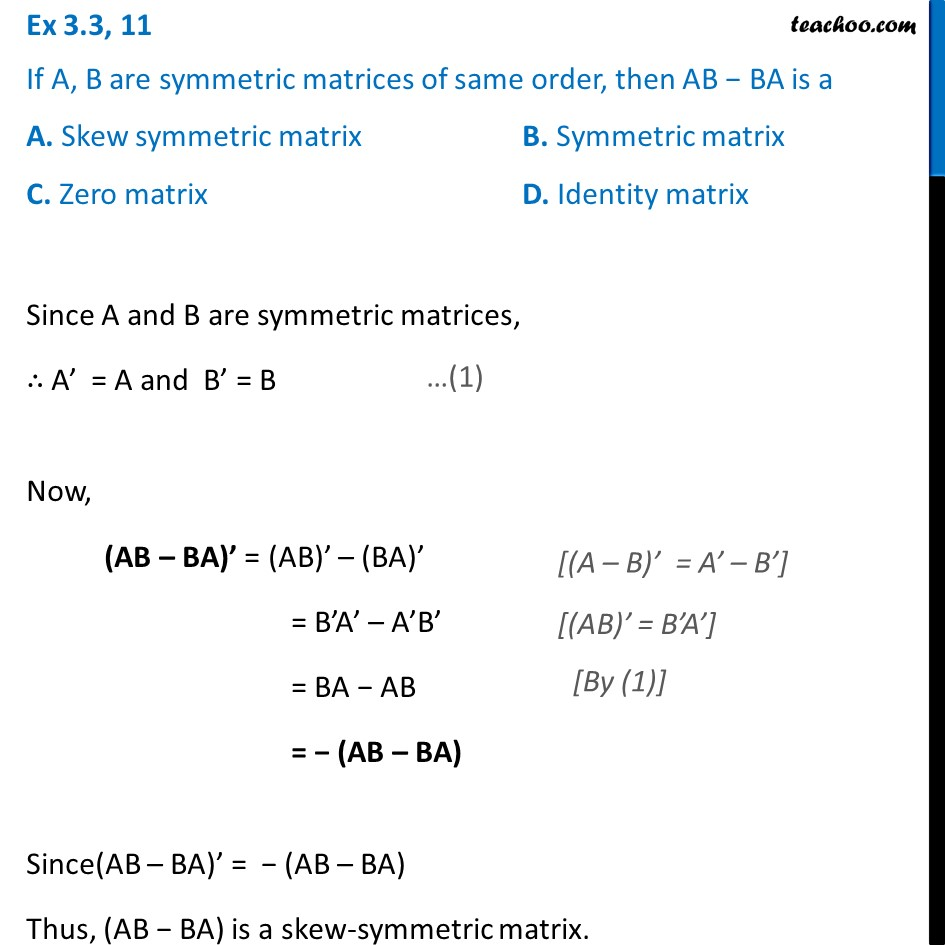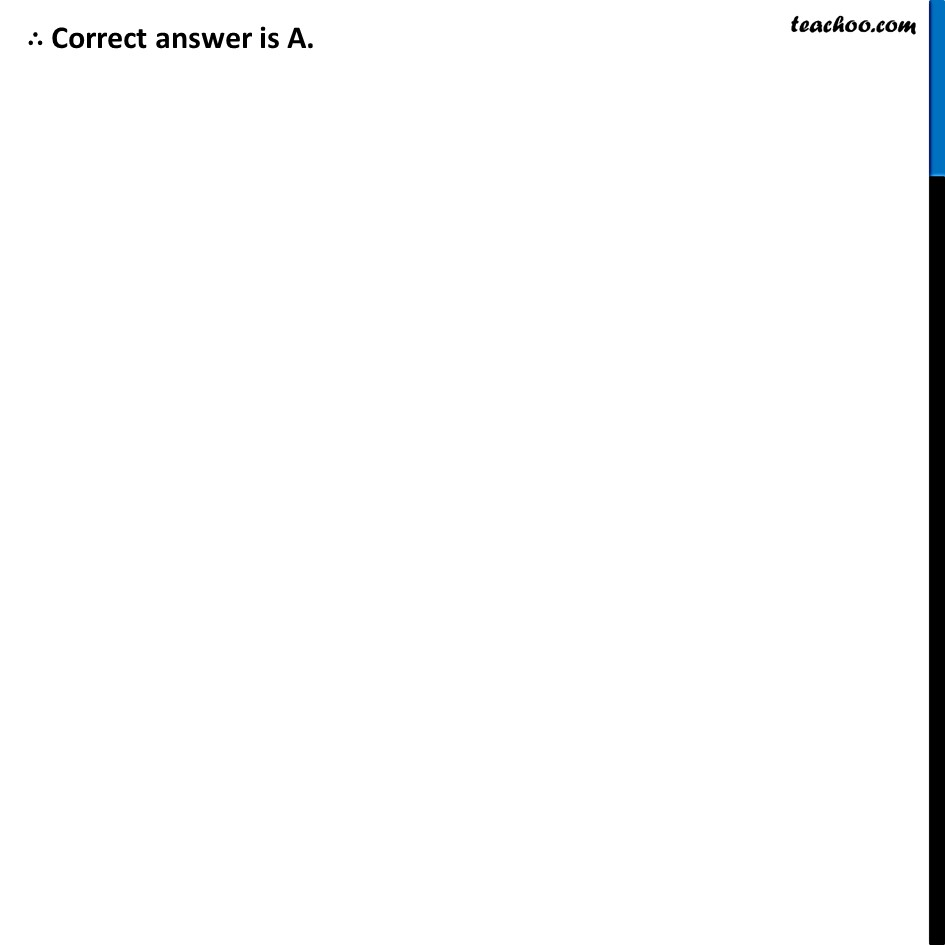Ex 3.3

Chapter 3 Class 12 Matrices
Serial order wiseLearn in your speed, with individual attention - Teachoo Maths 1-on-1 Class

### Transcript

Ex 3.3, 11 If A, B are symmetric matrices of same order, then AB − BA is a A. Skew symmetric matrix B. Symmetric matrix C. Zero matrix D. Identity matrix Since A and B are symmetric matrices, ∴ A’ = A and B’ = B Now, (AB – BA)’ = (AB)’ – (BA)’ = B’A’ – A’B’ = BA − AB = − (AB – BA) Since(AB – BA)’ = − (AB – BA) Thus, (AB − BA) is a skew-symmetric matrix. ∴ Correct answer is A. ∴ Correct answer is A.